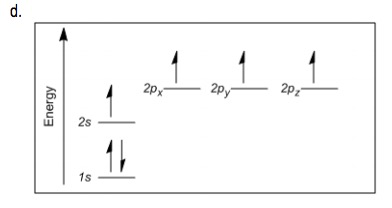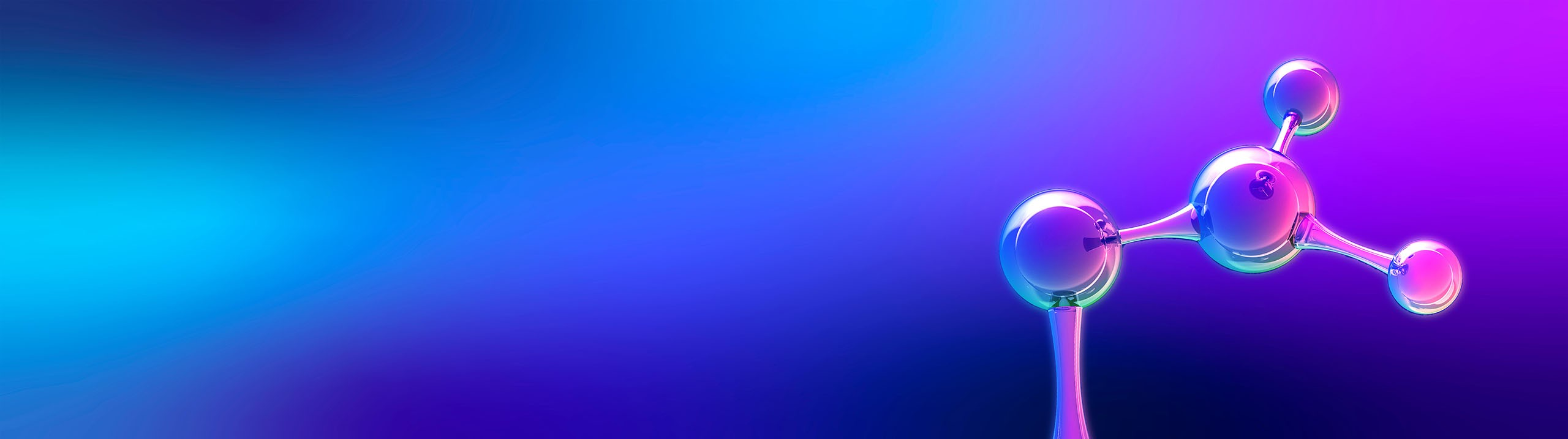## Organic Chemistry

Learn the toughest concepts covered in Organic Chemistry with step-by-step video tutorials and practice problems by world-class tutors.

1. A Review of General Chemistry

# Atomic Structure

Been awhile since Chem 1? Let’s cover some of the essentials from general chemistry that you’ll need for this course.

Recap of Protons and Neutrons
1
concept

## The difference between atomic numbers and atomic mass.1m
Play a video:
Hey everyone. So we're calling from general chemistry that the atom is the basic unit of matter and a collection of atoms is what helps to make a molecule. Now talking about adam we have an example of an atom in the top right corner here. Remember at the center of an atom is where we have a majority of the mass of the atom. That's where we have our protons and our neutrons here is not drawn to scale. The nucleus is very small here. We're just zooming in on it. So we can see the protons and the neutrons also remember that zooming around this are electrons which are found within different orbits. Now also remember that the atomic number of an atom is equal to the number of what That's right. Number of protons also remember that the atomic number is unique to a given element. So an element has atomic number and only that element has that atomic number. Besides the atomic number, we have the mass number. The mass number of an atom is equal to the number of protons plus neutrons. So we're talking about the total number of the subatomic particles with the nucleus of a particular atom. With this whole idea of protons, neutrons and electrons. We also know another term isotopes now, isotopes. These are a type of element that have the same number of protons and therefore the same atomic number but they have deferring neutrons. So their mass numbers is what's different from them. So they all have the same number of protons that it's just their mass numbers that are different. We'll go into further explanations for what exactly is an isotope and some examples in the following video.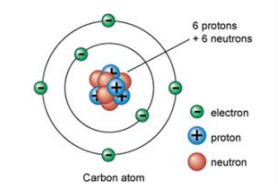Atomic Number: Number of protons in the atom.

Atomic Mass: Total number of protons and neutrons in the atom.

Isotopes: Atoms that have the same atomic number but differing atomic mass.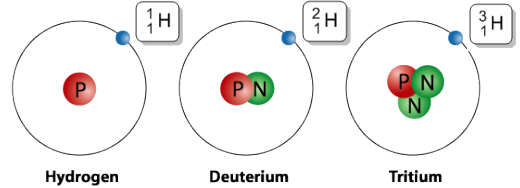2
example

## Understanding the hydrogen isotopes.1m
Play a video:
Recap of Electrons
3
concept

## Shells, orbitals and types of ions2m
Play a video:
• Shell: Region of space that electrons orbit around the nucleus in.
• Orbital: Region of space within a shell with exactly enough space for two electrons.
• Ion: An atom that has an unequal number of electrons and protons.
4
example

## Understanding the hydrogen ions.1m
Play a video:
So here's an example of some really good ions right here. So these are the simplest form of ions you can make, which would be the positive hydrogen and the negative ion of hydrogen. So let's talk about the positive one. Normally a hydrogen atom is a proton and an electron. If I take away one of those electrons, what's going to wind up happening is that I'm going to get a positive charge. Okay, I'm going to get a positively charged adam. And the reason is because I have nothing to counteract the positive charge of the of the, of the proton. All right now, when you have one of these H plus ions, it's actually just called a proton. Why? Because there's nothing else. There's no neutrons, there's no electrons. So literally we just call it protons. So when I say, hey, there's a proton whizzing whizzing around. That means it's a hydrogen that does not have an electron on it. Okay. And that means it has a positive charge. Then a hydride is the name of hydrogen that has a negative charge. Now, why would hydrogen ever have a negative charge? Well, if it has more than one electron for example, it has two electrons, then the electrons are gonna win. There's only more electrons and protons. So you have a net negative. So h minus is hydride H plus is a proton and you guys need to know that that's actually super important for organic chemistry
The Three Principles of Electron Configuration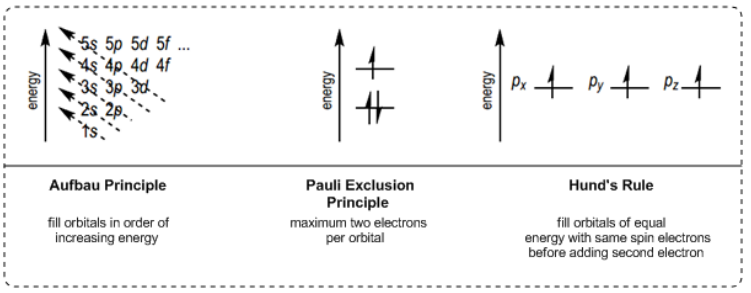5
concept

## Three rules about orbitals you need to know.2m
Play a video:
6
Problem

PRACTICE: Determine the number of protons, neutrons and electrons in the following atoms.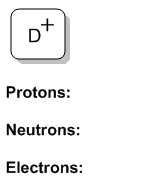7
Problem

PRACTICE: Determine the number of protons, neutrons and electrons in the following atoms.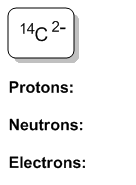8
Problem

PRACTICE: Determine which of the three principles of electron configuration is being broken in the electron diagrams below.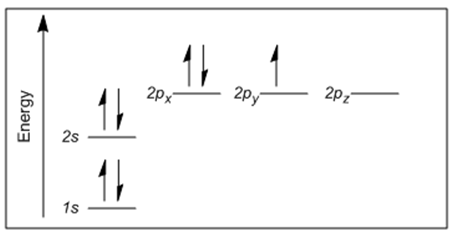9
Problem

PRACTICE: Determine which of the three principles of electron configuration is being broken in the electron diagrams below.